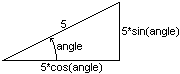### Sliding

Sliding is just what I call a animated movement or scrolling effect. By using looping (or iterating) functions and moving the layer in small increments, you can put together any sort of animated movement you can think of.

The basic idea is that you have your movement code:

```block.xpos += 5
block.left = block.xpos
```

Which moves the layer 5 pixels to the right. Then you stick that code into a looping function:

```function slide() {
if (block.xpos < 300) {
block.xpos += 5
block.left = block.xpos
setTimeout("slide()",30)
}
}
```

The if statement is there to determine when to stop the animation. In this case the function will stop when the x-position is at or over 300 pixels. The setTimeout() is what creates the loop. After a certain amount of milliseconds, it will execute whatever's inside the quotes. So this function will repeat itself every 30 milliseconds.

Of course it's not much more difficult to move on a diagonal - you just change both the xpos (left) and the ypos (top) values. Click here to see a diagonal example.

### Moving at a Given Angle

Using some high school trigonometry we can figure out how to move an element on any angle. In case you forgot, here's a quick diagram to refresh your memory:Now to initialize your object to include angles you'll need 4 new properties:

```object.angle = 30
object.xinc = 5*Math.cos(object.angle*Math.PI/180)
object.yinc = 5*Math.sin(object.angle*Math.PI/180)
object.count = 0
```

We calculate the x and y incrementation and use them to determine how far to move the left and top values. You have to multiply the angle by Math.PI/180 to convert the angle into radians - sin and cos are always calculated in radians. The count property will be used in the iterating function to determine how many times to loop.

Using my block example again, here's some full code to move an element at a given angle.

```function init() {
if (ns4) block = document.blockDiv
if (ie4) block = blockDiv.style
block.xpos = parseInt(block.left)
block.ypos = parseInt(block.top)
block.angle = 30
block.xinc = 5*Math.cos(block.angle*Math.PI/180)
block.yinc = 5*Math.sin(block.angle*Math.PI/180)
block.count = 0
}

function slide() {
if (block.count < 25) {
block.xpos += block.xinc
block.ypos -= block.yinc
block.left = block.xpos
block.top = block.ypos
block.count += 1
setTimeout("slide()",30)
}
else block.count = 0
}
```

The if (block.count < 25) means that the function will execute 25 times before stopping - ie4. the block will slide a total 125 pixels units.